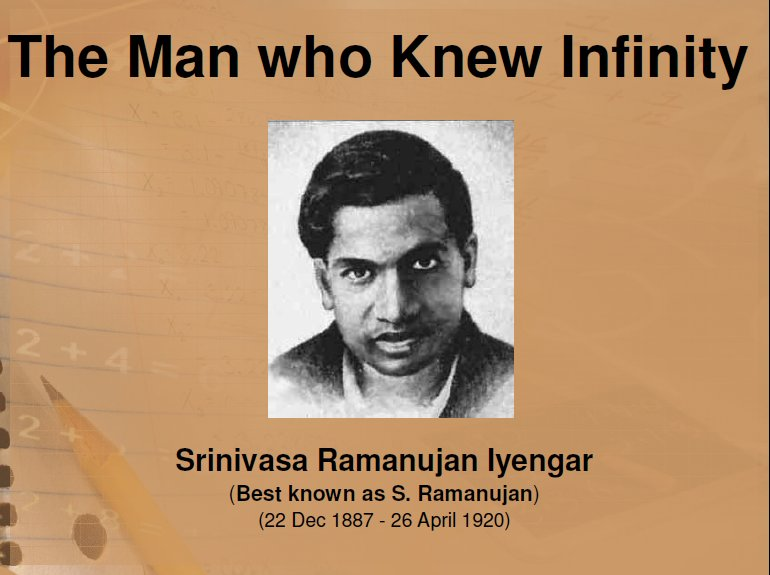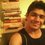# Lets use a Super-Calculator ! $e^{\pi\sqrt{163}}=$ Integer ?

To see if you qualify :- $(\text{Irrational})^{\text{Irrational}}=\text{Irrational} \\ \text{A. True} \quad \text{B. False} \quad \text{C. Unpredictable !}$

Ok. Fine ! Now what will be your reaction if you come out to know that $e^{\pi\sqrt{163}}$ is an integer. (waiting for your GIFs about your reaction). Don't you feel it strange that three irrational numbers $e$ , $\pi$ and $\sqrt{163}$ can be combined to form an integer ?

In fact, the Indian Mathematician Srinivasa Ramanujan (1888-1920) first conjectured that $e^{\pi\sqrt{163}}$ was an integer. He felt he found its value to be : $262537412640768743.999.......$In 1972, computers had carried it out to 2 million places of 9's, but to be an integer one must know it repeats forever.

So, if 9 repeats forever, then $e^{\pi\sqrt{163}}$ will be equal to $262537412640768744$

Now let me know if you're enough convinced to try to figure out its exact value !!!

Leave your amazing comments & ideas here. and Keep Exploring the Universe of Mathematics.Note by Sandeep Bhardwaj
6 years, 2 months ago

This discussion board is a place to discuss our Daily Challenges and the math and science related to those challenges. Explanations are more than just a solution — they should explain the steps and thinking strategies that you used to obtain the solution. Comments should further the discussion of math and science.

When posting on Brilliant:

• Use the emojis to react to an explanation, whether you're congratulating a job well done , or just really confused .
• Ask specific questions about the challenge or the steps in somebody's explanation. Well-posed questions can add a lot to the discussion, but posting "I don't understand!" doesn't help anyone.
• Try to contribute something new to the discussion, whether it is an extension, generalization or other idea related to the challenge.

MarkdownAppears as
*italics* or _italics_ italics
**bold** or __bold__ bold
- bulleted- list
• bulleted
• list
1. numbered2. list
1. numbered
2. list
Note: you must add a full line of space before and after lists for them to show up correctly
paragraph 1paragraph 2

paragraph 1

paragraph 2

[example link](https://brilliant.org)example link
> This is a quote
This is a quote
    # I indented these lines
# 4 spaces, and now they show
# up as a code block.

print "hello world"
# I indented these lines
# 4 spaces, and now they show
# up as a code block.

print "hello world"
MathAppears as
Remember to wrap math in $$ ... $$ or $ ... $ to ensure proper formatting.
2 \times 3 $2 \times 3$
2^{34} $2^{34}$
a_{i-1} $a_{i-1}$
\frac{2}{3} $\frac{2}{3}$
\sqrt{2} $\sqrt{2}$
\sum_{i=1}^3 $\sum_{i=1}^3$
\sin \theta $\sin \theta$
\boxed{123} $\boxed{123}$

Sort by:

I am astonishment! I NEVER knew that $\displaystyle { e }^{ \pi \sqrt { 163 } }$ could be an integer. I have so much to learn about mathematics. This is a perfect example of how simple intuition leads you astray. Can't trust your intuition, ya gotta do the math and work out all those decimals after the $...8743.999...$

Meanwhile, no, it's not true that ${ \left( Irrational \right) }^{ Irrational }=Irrational$ is always true. To see why, let $x$ be an unknown. Then we solve for $x$ the equation ${ x }^{ x }=2\quad$ or any other such rational number besides $2$. Then in nearly all cases, $x$ is not rational.

For a fascinating insight into this factoid, look up "Heegner Numbers" and check out Ramanujan's contribution to it.

- 6 years, 2 months ago

I am astonishment! I NEVER knew that eπ163√ could be an integer.

Is this sarcasm? Because it isn't an integer and Poe's law.

- 6 years, 2 months ago

Siddhartha, that was pretty cool, this is the first time I've heard of Poe's Law. It's right on target! Let me offer a corollary to Poe's Law, which is that no matter how outrageous or silly a politician's or pundit's statements may be, there will always be lots of people that believe them wholeheartedly.

Still, Heegner's numbers, to me, is a pretty fascinating subject. Heegner Number

Also here too Heegner Number

I really like the lineup of this very finite sequence of nine Heegner numbers

$1, 2, 3, 7, 11, 19, 43, 67, 163$

- 6 years, 2 months ago

What's the proof that there are only 9 of them?

- 6 years, 1 month ago

Look up Stark-Heegner Theorem What's interesting is that Carl Gauss as far back in the 19th century had conjectured that there would only be a finite number of them. But it took about a century to prove it, and another half century for other mathematicians to finally accept Heegner's 1952 proof of it, after it was upgraded by Stark in 1969. This has had a long history with a number of luminaries having worked on it, almost like Fermat's Last Theorem.

- 6 years, 1 month ago

That's interesting!

- 6 years, 1 month ago

Very interesting

- 6 years, 1 month ago

Wow!!

- 5 years, 11 months ago